Home » Comparative Stock Market Analysis in R using Quandl & tidyverse – Part I

# Comparative Stock Market Analysis in R using Quandl & tidyverse – Part I

## Introduction

What differentiates the best data scientists from others? It is their focus on application of data science. The best data scientists I know of, see data science and its application every where they look. They look at this world as an outcome of flow of data and information.

On the other hand, most beginners often ask the question – how do we apply our learning on real life problems?

In this post (and another one following this), I have picked up a real life dataset (Stock Markets in India) and showed how I would use this data to come out with useful insights.

I hope that you will find this useful. The idea is show the vast opportunities present in data science in a simple yet powerful manner. If you can think of more examples like this – let me know in comments below!

For the best results, I would strongly recommend to build the application yourself as you follow the tutorial.

## Objective of this Tutorial

In this article, we will analyze stock market in banking segment based on the bank stocks which are listed in NSE India. Our objective is to find the trends (Seasonal or cyclic) in banking stocks.

In our comparative analysis we will use several packages and the primary focus will be on tidy verse package. The emphasis will be given on grouping with the help of tibble dataframe from tidy verse package. This will help to perform similar operation on multiple groups at a time, hence reducing the code length and computational time.

This article also focuses on API Key, database code search using quandl, and finally how to directly download the data from R Console.

So lets get started!

Note: The code that has been mentioned below is to be run on the R command line for best results.

• Setting up the system
• Getting Started with Comparative Analysis
• Creating the dataset
• Visualizing the monthly prices
• Discovering the Relation between Total Traded Quantity vs Close Price
• Finding the Density Distribution of Deviation of High Price from Open Price
• Observing the Autocorrelation lags

## Setting Up The System

There are a few things you should take care of before you go on further. Below mentioned are the packages you need to install in the system

• timetk to coerce the dataframe into xts
• tidyverse to Use tibble for grouping and perform single operation on multiple groups
• tidyquant for Time Series and Financial functions to perform the analysis
• gglot for Plotting and Visualization
• forcats for modification of factor levels
• stringr for string use

If you don’t have any of the packages, then use the below code to install the packages. Modify the packages variable if any of the above packages are already installed.

``````pckgs<-c("Quandl","Sweep","tidyverse","tidyquant","ggplot","forcats","stringr")
install.packages(pckgs,dependencies = TRUE)``````

You can then call the necessary packages using the code below

``````library(Quandl)
library(tidyverse)
library(ggplot2)
library(tidyquant)
library(timetk)
library(forcats)
library(stringr)
library(gganimate)
library(plyr)
library(stringr)
library(gridExtra)``````

## Getting Started with Comparative Analysis

### Creating the Dataset

We will be using Quandl is online repository for the core financial, macroeconomic statistics and forex. Quandl has a vast collection of free and open data collected from a variety of organizations: central banks, governments, multinational organizations and more. You can use it without payment and with few restrictions.

Both Free and Premium data are available. Authenticated Free users have a limit of 300 calls per 10 seconds, 2,000 calls per 10 minutes and a limit of 50,000 calls per day. Premium data subscribers have a limit of 5,000 calls per 10 minutes and a limit of 720,000 calls per day.

We will use this online repository to get our data using “Quandl” package directly from the R Console. Quandl package directly interacts with the Quandl API to offer data in a number of formats usable in R, downloading a zip with all data from a Quandl database, and the ability to search.

To get started with Quandl, create an account and get the quandl API key. Please click here to create an account. Then click on the Login button provided on the top right corner of the screen. Once the registration is complete, please click here to get the API Key.

In our analysis, we have selected following banks

• ICICI
• BOB
• CANARA
• AXIS
• SBI
• PNB

We have selected these banks as they are in the price band of Rs 200 to Rs 500. We will use the following codes to get the data into R console.

`Quandl(Code=“NSE/—”,collapse=“—”,start_date=“—-”,type=“…”)`

The parameters we use are as follows:

• Code Dataset code on Quandl specified as a string or an array of strings.
• collapse Collapse frequency of Data.Eg; “daily”, “monthly”, “weekly”, “yearly”.
• start_date Desired Start Date
• type Type of data returned specified as string. Can be ‘raw’, ‘ts’, ‘zoo’, ‘xts’ or ‘timeSeries’

Now we will download the data, add a column “Stock” for the stock identifier, and then we paste the respective stock name in the downloaded dataset. We will then consolidate all stock data into one Master Data frame for analysis

``````## Setup the Quandl Free Account and API Key, Please copy and paste the API key in order to #authenticate

Quandl.api_key("<Your-API-Key>")

ICICI = Quandl("NSE/ICICIBANK",collapse="daily",start_date="2016-09-01",type="raw")
PNB= Quandl("NSE/PNB",collapse="daily",start_date="2016-09-01",type="raw")
Axis=Quandl("NSE/AXISBANK",collapse="daily",start_date="2016-09-01",type="raw")
Canara=Quandl("NSE/CANBK",collapse="daily",start_date="2016-09-01",type="raw")
BOB=Quandl("NSE/BANKBARODA",collapse="daily",start_date="2016-09-01",type="raw")
SBI=Quandl("NSE/SBIN",collapse="daily",start_date="2016-09-01",type="raw")

## Add another ("Stock") coloumn in Datasets using cbind command

ICICI<-cbind(ICICI,Stock="")
PNB<-cbind(PNB,Stock="")
Axis<-cbind(Axis,Stock="")
SBI<-cbind(SBI,Stock="")
Canara<-cbind(Canara,Stock="")
BOB<-cbind(BOB,Stock="")

## Paste the stock name in stock column

ICICI\$Stock<-paste(ICICI\$Stock,"ICICI",sep="")
PNB\$Stock<-paste(PNB\$Stock,"PNB",sep="")
Axis\$Stock<-paste(Axis\$Stock,"Axis",sep="")
SBI\$Stock<-paste(SBI\$Stock,"SBI",sep="")
Canara\$Stock<-paste(Canara\$Stock,"Canara",sep="")
BOB\$Stock<-paste(BOB\$Stock,"BOB",sep="")

## Consolidate under one dataset

Master_Data<-rbind(ICICI,PNB,Axis,SBI,Canara,BOB)
``````

### Visualization of monthly prices

Let us look at Monthly and Daily price pattern for Stocks using ggplot package. For this we will need to group the master dataframe according by Stock.

We have heavily manipulated the theme section of ggplot to get the desired plot. More information on plot is provided here.

``````## Visualisation in ggplot2 ("Comparative Visulisation of Close Price listed on NSE")

## Convert the dates into character in order to split the coloumn into "Y" "m" "dd"" columns
Master_Data\$Date<-as.character(Master_Data\$Date)

## Split the date and create a list for the same

list<-strsplit(Master_Data\$Date,"-")

## Convert the list into dataframe
library(plyr)
Master_Date1<-ldply(list)
colnames(Master_Date1)<-c("Year","Month","Day")

## Column bind with the main dataframe
Master_Data<-cbind(Master_Data,Master_Date1)
names(Master_Data)

## Change the scale for Traded Quantity

## Convert the Date to as.Date()

Master_Data\$Date<-as.Date(Master_Data\$Date)

## Visualisation with Bubble Plot
P<- ggplot(Master_Data,aes(factor(Stock),Close,color=Stock,frame=Month)) +
geom_jitter(aes(size = Close, colour=Stock, alpha=.02)) +
ylim(0,1000)+
labs(title = "Bank Stock Monthly Prices", x = "Banks", y= "Close Price") +
theme(panel.border = element_blank(),
panel.grid.major = element_line(colour = "grey61", size = 0.5, linetype = "dotted"),
panel.grid.minor = element_blank(),
axis.line=element_line(colour="black"),
plot.title = element_text(hjust = 0.5,size=18,colour="indianred4"))+
theme(legend.position="none")

P1<-gganimate(P,'Price_Range.gif',ani.width=600,ani.height=400,interval=1)

## Group By Stock

Master_Data<-Master_Data%>%
tibble::as.tibble()%>%
group_by(Stock)

## Visualisation for Daily Stock Prices

Master_Data %>%
ggplot(aes(x = Date, y = Close, color = Stock)) +
geom_point() +
labs(title = "Daily Close Price", x = "Month",y="Close Price") +
facet_wrap(~ Stock, ncol = 3, scale = "free_y") +
scale_fill_tq(fill="green4",theme="light") +
theme_tq() +
theme(panel.border = element_blank(),
panel.grid.major = element_line(colour = "grey61", size = 0.5, linetype = "dotted"),
panel.grid.minor = element_blank(),
axis.line=element_line(colour="black"),
plot.title = element_text(hjust = 0.5,size=18,colour="indianred4"))+
theme(legend.position="none")

``````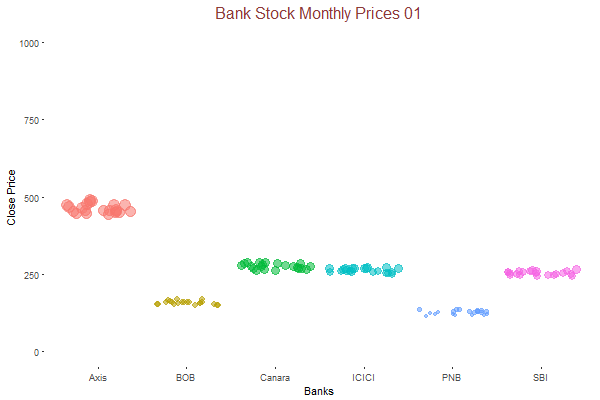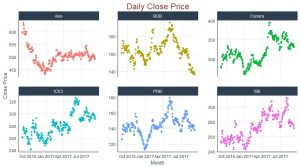### Discovering the Relation between Total Traded Quantity vs Close Price

Usually, traded quantity increases if the stock price increases or decreases too rapidly on a given day. This parameter is important for our model for prediction. So we should take some time out to identify the relation between them in our data.

``````## Traded Quantity vs Price

z<-Master_Data %>%
ggplot(aes(x = `Total Trade Quantity`, y = Close, color = Stock,frame=Month)) +
geom_smooth(method='loess') +
xlim(0,400)+
labs(title = "Monthly Traded Quantity vs Price", x = "Traded Quantity (Lacs)",y="Close Price") +
facet_wrap(~ Stock, ncol = 3, scale = "free_y") +
scale_fill_tq(fill="green4",theme="light") +
theme_tq() +
theme(panel.border = element_blank(),
panel.grid.major = element_blank(),
panel.grid.minor = element_blank(),
plot.title = element_text(hjust = 0.5,size=18,colour="indianred4"),
axis.line = element_line(colour = "black"))+
theme(legend.position="none")

z1<-gganimate(z,'Quantity_Price.gif',ani.width=600,ani.height=400,interval=0.7)

``````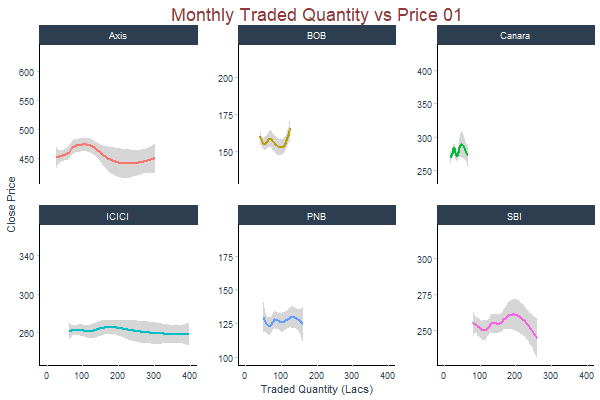We have an idea of trend of the stock price, but not much is clear from the Monthly prices. Axis Bank share price improved in september and stayed at Rs750 for a month. whereas all other Banks were consistent and did not show much of volatility.

### Finding the Density Distribution of Deviation of High Price from Open Price

Now we will see the density distribution of High Price from Open Price in order to get an understanding that how much price is deviating in either direction (North or South) on weekly basis. This gives us an idea of price range for any stock in intraday trading.

For this add a new column with the difference of high and open price using mutate function. Add another new column with the difference of low and open price using mutate function. Calculate the weekly average of differences using “tq_transmute()” function from tidyverse package. Visualize both density plots with dot distribution on ggplot

```## Deviation from High & Low Price
Master_Data_High<-Master_Data%>%mutate(Dev_High=High-Open)
Master_Data_Low<-Master_Data%>%mutate(Dev_Low=Open-Low)

## Computation of weekly average for high Price

Master_Data_High_Week <- Master_Data_High %>%
tq_transmute(
select     = Dev_High,
mutate_fun = apply.weekly,
FUN        = mean,
na.rm      = TRUE,
col_rename = "Dev_High_Mean"
)

## Computation weekly average for Low Price

Master_Data_Low_Week<-Master_Data_Low%>%
tq_transmute(
select  = Dev_Low,
mutate_fun = apply.weekly,
FUN = mean,
na.rm = TRUE,
col_rename = "Dev_Low_Mean"
)

## Visualisation of density distribution of High Price

High<-Master_Data_High_Week%>%ggplot(aes(x=Dev_High_Mean,color=Stock))+
geom_dotplot(binwidth=0.50,aes(fill=Stock))+
xlim(0,10)+
scale_fill_manual(values=c("#999999", "#E69F00","#CC9933","#99FF00","#CC3399","#FF9933"))+
labs(title="Distribution of High Price Deviation from Open Price",x="Weekly Mean Deviation")+
facet_wrap(~Stock,ncol=3,scale="free_y")+
scale_color_tq(values=c("#999999"))+
theme_tq()+
theme(panel.border = element_blank(),
panel.grid.major = element_line(colour = "grey61", size = 0.5, linetype = "dotted"),
panel.grid.minor = element_blank(),
axis.line=element_line(colour="black"),
plot.title = element_text(hjust = 0.5,size=16,colour="indianred4"))+
theme(legend.position="none")

## Visualisation of density distribution of Low Price
Low<-Master_Data_Low_Week%>%ggplot(aes(x=Dev_Low_Mean,color=Stock))+
geom_dotplot(binwidth=0.50,aes(fill=Stock))+
xlim(0,10)+
scale_fill_manual(values=c("#999999", "#E69F00","#CC9933","#99FF00","#CC3399","#FF9933"))+
labs(title="Distribution of Weekly Low Price Deviation from Open Price",x="Weekly Mean Deviation")+
facet_wrap(~Stock,ncol=3,scale="free_y")+
scale_color_tq(values=c("#999999"))+
theme_tq()+
theme(panel.border = element_blank(),
panel.grid.major = element_line(colour = "grey61", size = 0.5, linetype = "dotted"),
panel.grid.minor = element_blank(),
axis.line=element_line(colour="black"),
plot.title = element_text(hjust = 0.5,size=16,colour="indianred4"))+
theme(legend.position="none")
## Arrange
grid.arrange(High,Low,ncol = 2, nrow = 1)```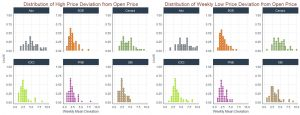### Observing the Autocorrelation lags

The lag operator (also known as backshift operator) is a function that shifts (offsets) a time series such that the “lagged” values are aligned with the actual time series. The lags can be shifted any number of units, which simply controls the length of the backshift.

Here, “k” is denoted as lag. We will see the lag of 180 days period and see how stocks behave.

These are the steps for Computation

• Define k lag period
• Create columns for lag periods
• Group the data by Stock by creating new data frame for lags
• Apply lag.xts using tq_mutate() function on the new dataframe
• Apply Auto-correaltion
```k <- 1:180
col_names <- paste0("lag_", k)

## Only Select Columns "Date" and "Close" from hte master data frame.

Master_Data_lags<-Master_Data%>%
tibble::as_tibble() %>%
group_by(Stock)

Master_Data_lags<-Master_Data_lags%>%select(Date,Close)
# Apply lag.xts function using tq_mutate

Master_Data_lags<-Master_Data_lags%>%
tq_mutate(
select = Close,
mutate_fun = lag.xts,
k=1:180,
col_rename=col_names
)

# Calculate the autocorrelations and 95% cutoffs

Master_Data_AutoCorrelations<-Master_Data_lags %>%
gather(key = "lag", value = "lag_value", -c(Stock,Date, Close)) %>%
mutate(lag = str_sub(lag, start = 5) %>% as.numeric) %>%
group_by(Stock, lag) %>%
summarize(
cor = cor(x = Close, y = lag_value, use = "pairwise.complete.obs"),
cutoff_upper = 2/(n())^0.5,
cutoff_lower = -2/(n())^0.5
)

## Visualisation of Autocorrelation: ACF Plot

Master_Data_AutoCorrelations %>%
ggplot(aes(x = lag, y = cor, color = Stock, group = Stock)) +

# Add horizontal line a y=0
geom_hline(yintercept = 0) +

# Plot autocorrelations
geom_point(size = 2) +
geom_segment(aes(xend = lag, yend = 0), size = 1) +

geom_line(aes(y = cutoff_upper), color = "blue", linetype = 2) +
geom_line(aes(y = cutoff_lower), color = "blue", linetype = 2) +

facet_wrap(~ Stock, ncol = 3) +

# Aesthetics
expand_limits(y = c(-1, 1)) +
scale_color_tq() +
theme_tq() +
labs(
title = paste0("Tidyverse ACF Plot: Lags ", rlang::expr_text(k)),
x = "Lags"
) +
theme(
legend.position = "none",
axis.text.x = element_text(angle = 45, hjust = 1),
panel.grid.major = element_line(colour = "grey61", size = 0.5, linetype = "dotted"),
plot.title = element_text(hjust = 0.5,size=18,colour="indianred4")
)```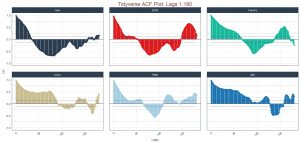Its apparent from the ACF plot, that there is no weekly or monthly pattern.

## End Notes

This article contains descriptive analysis of stocks in terms of Daily/Weekly Price fluctuations. It also includes analysis on deviation from High and Low Price. The focus is also given on the relationship between the daily traded quantity of shares & close price and to check for the relationship. In the later part, the main focus is on xts package for the computation of Auto-correaltion. In the article, the focus is provided on finding lag and acf plot using ggplot rather than using the conventional time series package. This includes the analysis on ACF using different lags and to check if there is any pattern in the series.Aritra Chatterjee is a professional in the field of Data Science and Operation Management having experience of more than 5 years. He aspires to develop skill in the field of Automation, Data Science and Machine Learning.

### Learn, engage,compete, and get hired!

You can also read this article on our Mobile APP•sharad says:

Thanks a lot Aritra, very nice artical . Good information.

•Aritra Chatterjee says:

•richie31 says:

•Vikram says:

Hi, My API Code for Quandl doesn’t seem to work. Can you please suggest might be wrong????

•richie31 says:

Hi Vikram,

It should work, provided you are using your own key after creating an account with quandl.

•pavan says:

Its really a good stuff. thanks for sharing

•richie31 says:

Thank you, Pavan.

•san says:

Eagerly waiting for part II , any tentative dates for the same?

•richie31 says:

Probably by the last week of this month.

•amit says:

Not able to install ggplot and gganimate in Rstudio .Getting the error message as ” package ‘gganimate’ is not available (for R version 3.4.1) “.While my Rstudio is installed with latest version.

•amit says:

RStudio
Version 1.0.153 – © 2009-2017 RStudio, Inc.

•richie31 says:

Hello Amit,

Please use the below code to install directly from github,

Note: Your system must have Image Magick Software. Please install devtools package in R.
library(devtools)
install_github(“dgrtwo/gganimate”)

•Amaresh says:

Nice explanation.
But I am not able to execute any gganimate commands such as
P1<-gganimate(P,'Price_Range.gif',ani.width=600,ani.height=400,interval=1)

I am receiving the below error:

I cannot find ImageMagick with convert = "convert"
but I can find it from the Registry Hive: C:\Program Files\ImageMagick-7.0.7-Q16
Executing:
"C:\Program Files\ImageMagick-7.0.7-Q16\convert.exe -loop 0 -delay 100
Rplot1.png Rplot2.png Rplot3.png Rplot4.png Rplot5.png Rplot6.png
Rplot7.png Rplot8.png Rplot9.png Rplot10.png Rplot11.png
Rplot12.png "Price_Range.gif""
'C:\Program' is not recognized as an internal or external command,
operable program or batch file.
an error occurred in the conversion… see Notes in ?im.convert
Error in file(file, "rb") : cannot open the connection

Need some suggestions

•richie31 says:

Hello Amaresh,

I forgot to mention to load the “animation” package in order to use the “ani.width” and “ani.height” features.
Please see my previous post on gganimate to get more details on the same,

•sv says:

•Amit says:

Getting error message (I already installed ImageMagic in my pc as well as in R using install.packages(“magick”)) Still getting below error message .So please let me know where the things are getting wrong?

P1

•richie31 says:

Hi Amit,

P1 is the variable. You either enter P1 in console or run it directly without storing it in variable. Please let me know if this help.

•Akash says:

Hello. I could not find the link to the second part of this analysis which has Predictive model.

•Aishwarya Singh says:

Hi Akash,

Here is the link you are looking for.

•Akash says:

Thank you !